Question

# Use ordinary interest: Principal \$70,000 Rate of Interest: 11% Time: 90 days Maturity Value: A Date note made Mar 10 Date note discounted: April 15 discount period: B proceeds: C note to be discounted at 10%

Use ordinary interest: Principal \$70,000 Rate of Interest: 11% Time: 90 days Maturity Value: A Date note made Mar 10 Date note discounted: April 15 discount period: B proceeds: C note to be discounted at 10%

We need at least 6 more requests to produce the answer.

4 / 10 have requested this problem solution

The more requests, the faster the answer.

All students who have requested the answer will be notified once they are available.

#### Earn Coins

Coins can be redeemed for fabulous gifts.

Similar Homework Help Questions
• ### what is the discount period, bank discount, and proceeds on 25000.00 at 9% for 60 days, date of note is June 8 and date note discounted is July 10

what is the discount period, bank discount, and proceeds on 25000.00 at 9% for 60 days, date of note is June 8 and date note discounted is July 10?

• ### Actuarial Science Mathematics of Finance Discounted Value 6. For cach promissory note in the following table, determine the maturity date, maturity value, dis- counted period, and the proceeds. No.Da...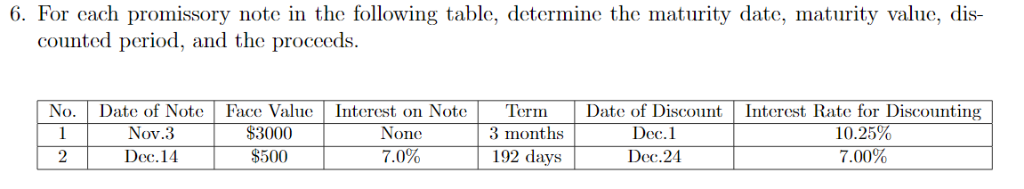Actuarial Science Mathematics of Finance Discounted Value 6. For cach promissory note in the following table, determine the maturity date, maturity value, dis- counted period, and the proceeds. No.Date of Note Face ValueInterest on NoteTermDate of Discount Interest Rate for Discounting Nov.3 \$3000 None Dec.1 3 months \$500 Dec.24 192 days 7.00% 2 Dec.14 7.0% 6. For cach promissory note in the following table, determine the maturity date, maturity value, dis- counted period, and the proceeds. No.Date of Note Face...

• ### Solve for maturity value, discount period, bank discount, and proceeds.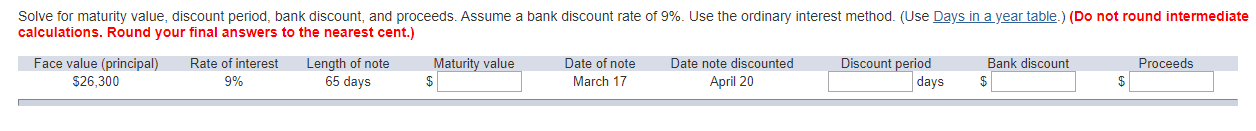Solve for maturity value, discount period, bank discount, and proceeds. Assume a bank discount rate of 9%. Use the ordinary interest method. (Use Days in a year table.) (Do not round intermediate calculations. Round your final answers to the nearest cent.)

• ### Compute interest and find the maturity date for the following notes. (Use 360 days for calculation.)...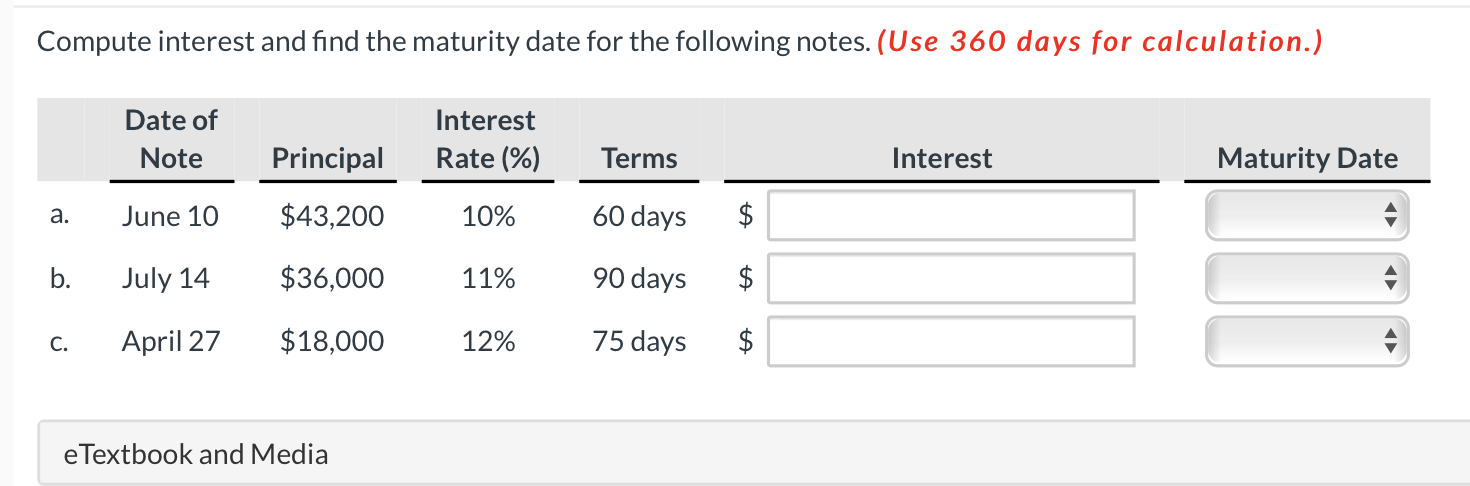Compute interest and find the maturity date for the following notes. (Use 360 days for calculation.) Date of Note Interest Rate (%) Principal Terms Interest Maturity Date a. June 10 \$43,200 10% 60 days \$ b. July 14 \$36,000 11% 90 days \$ C. April 27 \$18,000 12% 75 days \$ \$ e Textbook and Media

• ### Determine the maturity date and compute interest for each note. (Use 360 days a year. Do...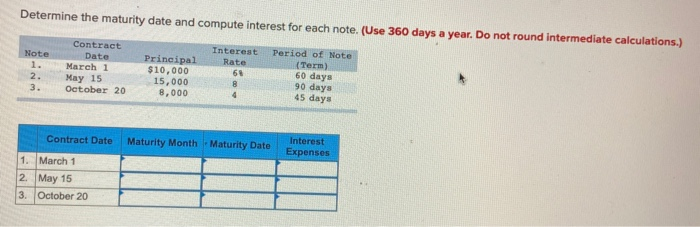Determine the maturity date and compute interest for each note. (Use 360 days a year. Do not round intermediate calculations.) Note Contract Date March 1 May 15 October 20 Principal \$10,000 15,000 8,000 Interest Period of Note Rate (Term 60 days 90 days 45 days Contract Date Maturity Month - Maturity Date Interest Expenses 1. March 1 2. May 15 3. October 20

• ### 7 Homework Saved Determine the maturity date and compute interest for each note. (Use 360 days...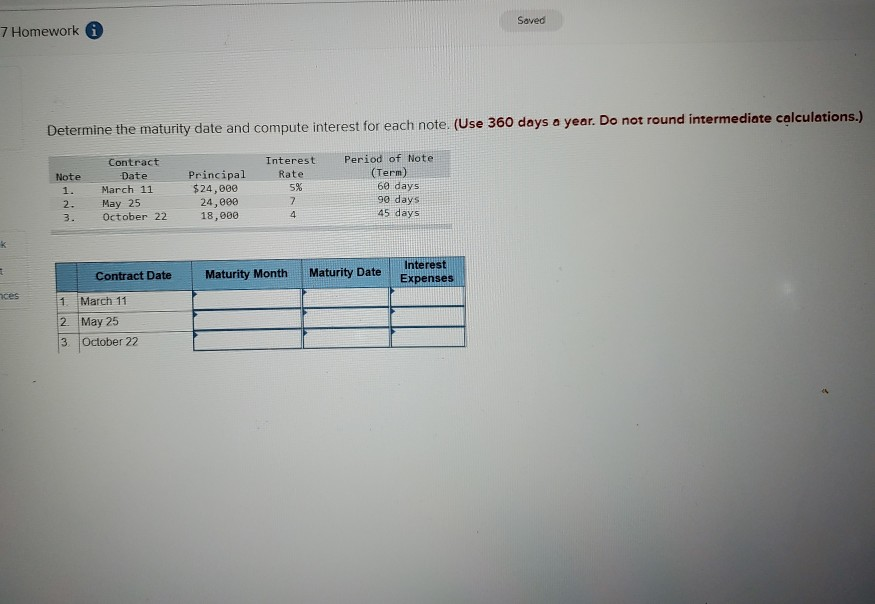7 Homework Saved Determine the maturity date and compute interest for each note. (Use 360 days a year. Do not round intermediate calculations.) Note Contract Date March 11 May 25 October 22 Interest Rate 5% Principal \$24,000 24,000 18,000 Period of Note (Term) 60 days 90 days 45 days 2. Contract Date Maturity Month Maturity Date Sate Interest Expenses ces 1 March 11 2 May 25 3 October 22

• ### Determine the maturity date and compute interest for each note. (Use 360 days a year. Do...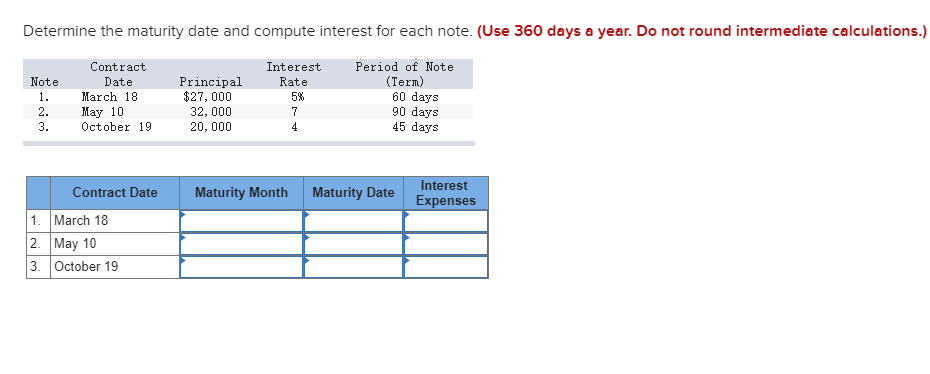Determine the maturity date and compute interest for each note. (Use 360 days a year. Do not round intermediate calculations.) Note Contract Date March 18 May 10 October 19 Interest Rate 5% 1. Period of Note (Term) 60 days 90 days 45 days Principal \$27,000 32,000 20,000 2. 3. Maturity Month Maturity Date Interest Expenses Contract Date 1. March 18 2. May 10 3. October 19

• ### Determine the maturity date and compute interest for each note. (Use 360 days a year. Do...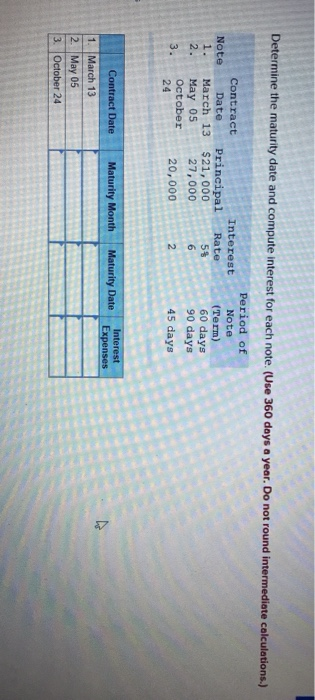Determine the maturity date and compute interest for each note. (Use 360 days a year. Do not round intermediate calculations.) Note 1. Interest Rate Contract Date Principal March 13 \$21,000 May 05 27,000 October 20,000 5\$ Period of Note (Term) 60 days 90 days 45 days 24 Maturity Month Maturity Date Interest Expenses Contract Date 1. March 13 2. May 05 3. October 24

• ### Determine the maturity date and compute interest for each note. (Use 360 days a year. Do...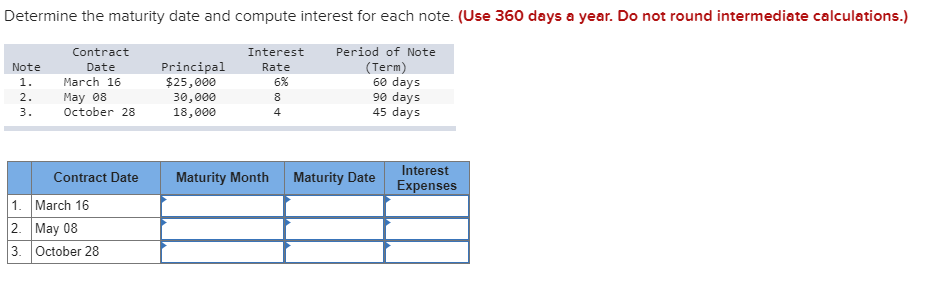Determine the maturity date and compute interest for each note. (Use 360 days a year. Do not round intermediate calculations.) Period of Note Contract Interest Principal \$25,000 (Term) 60 days 90 days 45 days Note Date Rate March 16 6% 1. May 08 October 28 2. 30,000 18,000 8 3. 4 Interest Maturity Month Maturity Date Contract Date Expenses 1. March 16 2. May 08 3 October 28

• ### Determine the maturity date and compute interest for each note. (Use 360 days a year. Do...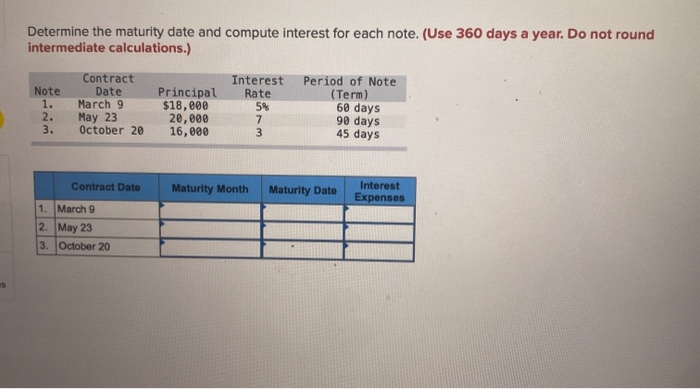Determine the maturity date and compute interest for each note. (Use 360 days a year. Do not round intermediate calculations.) Note 1. 2. 3. Contract Date March 9 May 23 October 20 Principal \$18,000 20,000 16,000 Interest Rate 5% 7 3 Period of Note (Term) 60 days 90 days 45 days Contract Date Maturity Month Maturity Dato Interest Expenses 1. March 9 2. May 23 3. October 20